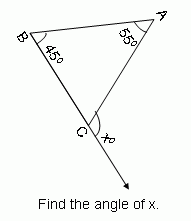Email us to get an instant 20% discount on highly effective K-12 Math & English kwizNET Programs!

#### Online Quiz (WorksheetABCD)

Questions Per Quiz = 2 4 6 8 10

### Geometry2.8 Theorem: Exterior Angle Theorem of a Triangle

 Exterior Angle Theorem: The measure of an exterior angle of a triangle is equal to the sum of the measures of the two nonadjacent interior angles.Directions: Solve the following problems. Also draw a triangle and label its interior and exterior angles and find the measure of all the 6 exterior angles.
 Q 1:110 degrees60 degrees50 degrees Q 2:100 degrees45 degrees55 degrees Q 3:115 degrees55 degrees60 degrees Q 4:55 degrees100 degrees45 degrees Question 5: This question is available to subscribers only! Question 6: This question is available to subscribers only!